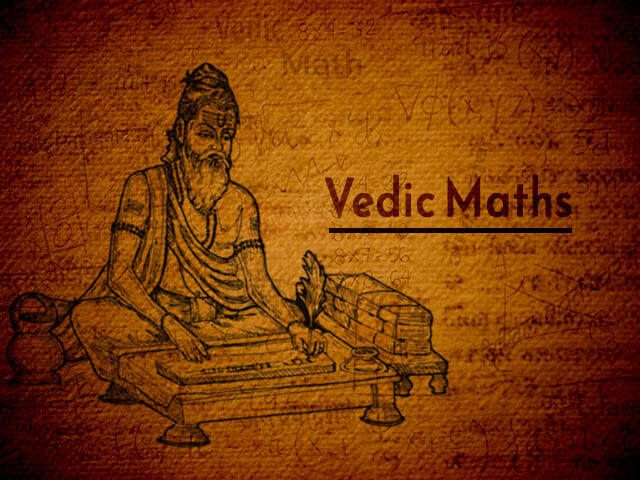# Unlocking the Secrets of Vedic Mathematics: How to Improve Your Mental Calculation Skills

Mental calculation, or the ability to perform calculations in your head, is an essential skill for mathematicians, scientists, and even everyday people. It's not just about quick arithmetic, but also about a deeper understanding of numbers and mathematical concepts. Vedic mathematics, an ancient Indian system of calculation, has long been touted as an effective way to improve mental calculation skills. In this post, we'll explore how Vedic mathematics can help you improve your mental math abilities.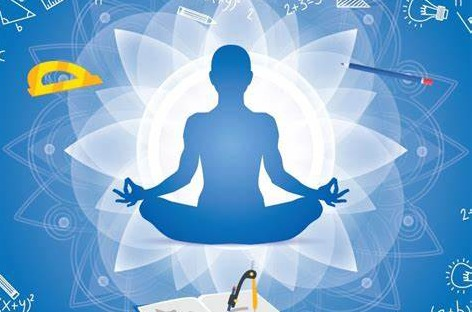One of the key principles of Vedic mathematics is the use of Sutras, or formulas that can be used to quickly solve mathematical problems. These sutras are based on ancient Indian wisdom and are designed to be easy to memorize and use. One such sutra, "Ekadhikina Purvena" (By one more than the previous one), is a method of addition that utilizes the place value system of numbers, making it easy to perform addition in your head. Another key principle of Vedic mathematics is the emphasis on visualization. Instead of seeing numbers as abstract concepts, Vedic mathematics encourages us to see them as objects that can be manipulated and transformed. This can make it easier to perform mental calculations because you are able to "see" the numbers in your head.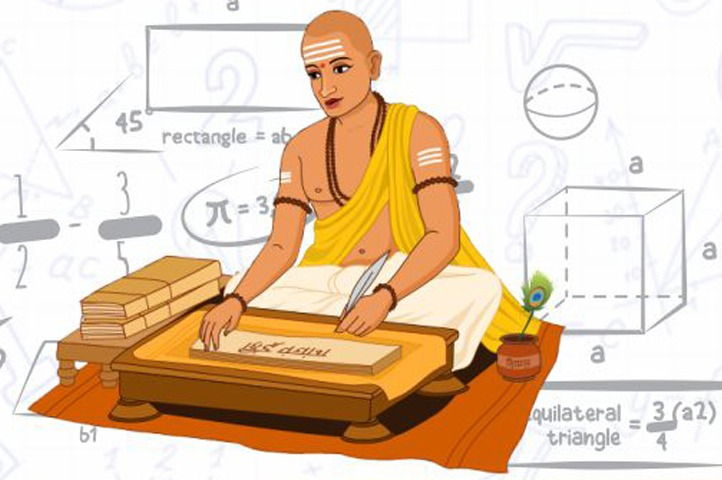In addition to Sutras and visualization, Vedic mathematics also emphasizes speed. The goal of Vedic mathematics is to perform calculations quickly and accurately, and the system is designed to help you achieve this goal. This can be done through the use of shortcuts, mental math tricks, and memory techniques that can be practiced and mastered over time. As I started learning Vedic mathematics, I was initially sceptical about its ability to improve my mental calculation skills. But as I began to dive deeper into the system, I quickly discovered that it was not only effective but also fun to learn. One of the first techniques I learned was "Ekadhikina Purvena", and I was amazed at how quickly I could perform addition using this method. I found myself easily doing calculations that I previously thought required a calculator.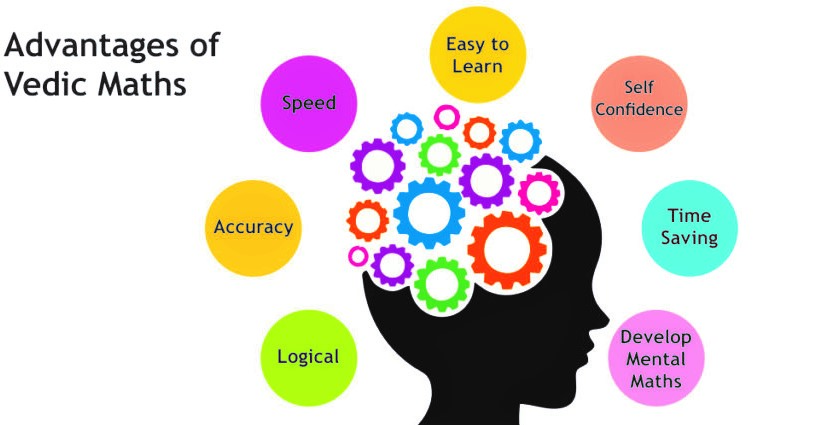I also found that visualization was an important aspect of Vedic mathematics, which made it much easier to perform mental calculations. Instead of seeing numbers as abstract concepts, I began to see them as objects that could be transformed and manipulated. This made it much easier to perform mental math, as I could "see" the numbers in my head. As I continued to practice and improve my Vedic mathematics skills, I noticed that my overall understanding of mathematics was also improving. I felt like I had a better understanding of how numbers work, and I felt more confident in my ability to solve mathematical problems. And it was not only about math it helped me in many areas like problem solving, thinking in terms of patterns and being more present in the moment.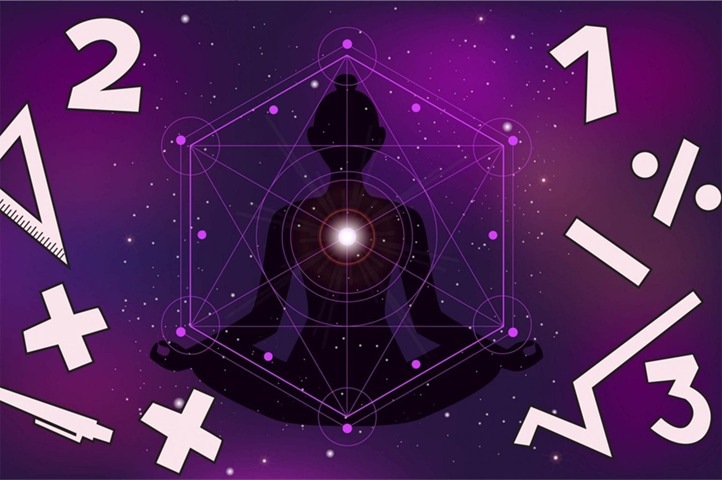I would highly recommend Vedic mathematics to anyone looking to improve their mental calculation skills. It's a fun and engaging system that can help you become a better problem solver, and it's an excellent way to develop a deeper understanding of mathematics. Finally, Vedic mathematics is also an excellent way to develop a deeper understanding of mathematics. By learning and practicing Vedic mathematics techniques, you can gain a new perspective on numbers and mathematical concepts, and a better understanding of how mathematics works.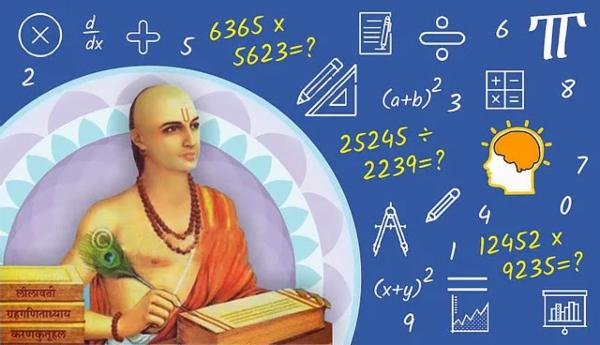In conclusion, Vedic mathematics is an ancient Indian system of calculation that can be used to improve mental math abilities. It offers various techniques and formulas to perform mental calculations more quickly and accurately. Through visualization, speed and a deeper understanding of mathematics, Vedic mathematics can help to improve problem solving skills.

### Author